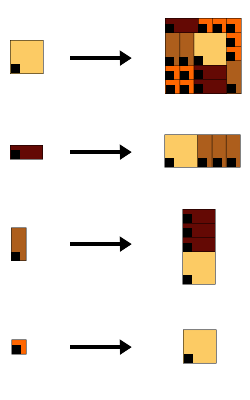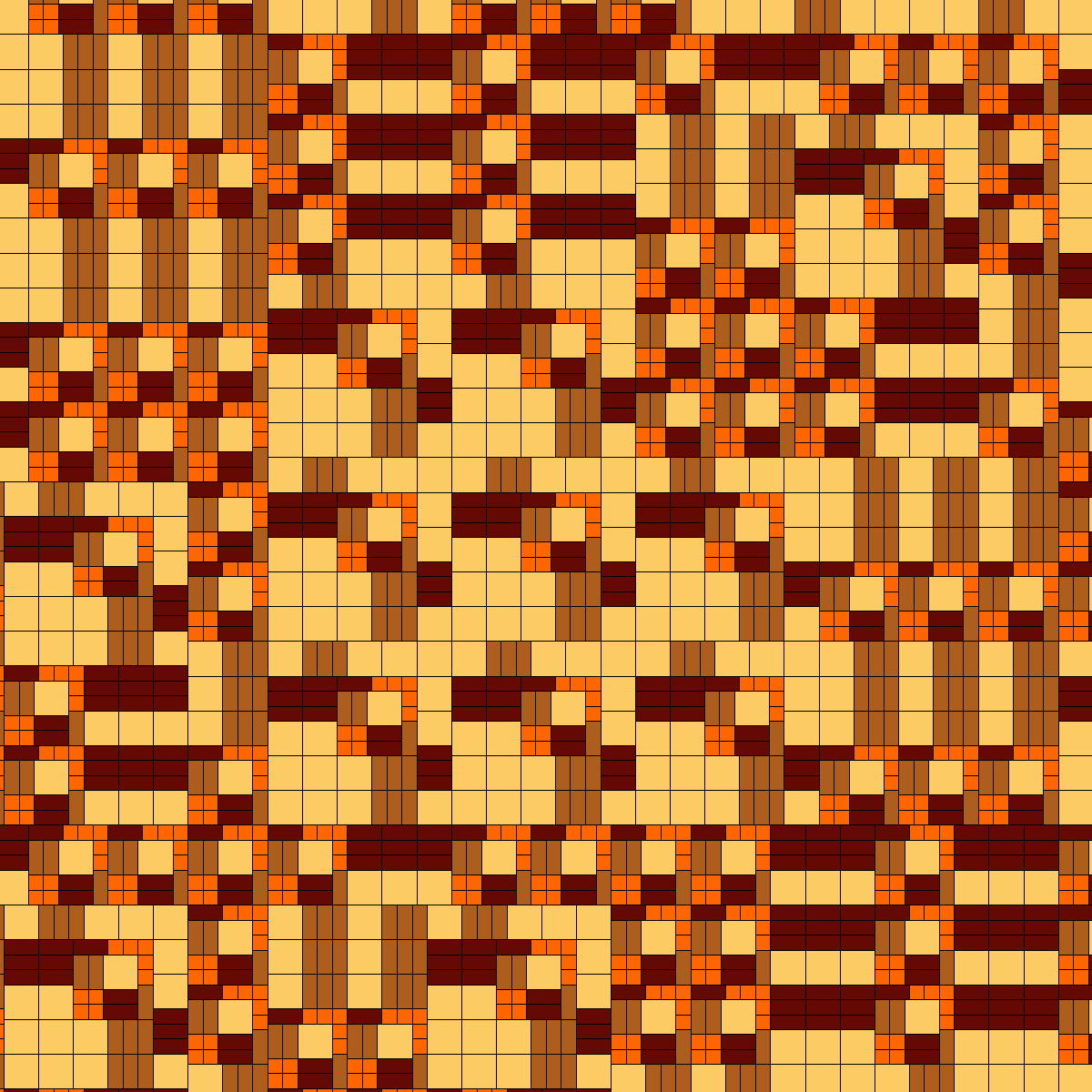## Priebe Frank non PV

### Info

A plane substitution tiling which does not possess flc. It arises from the 1-dimensional substitution a -> abbb, b -> a, which inflation factor is not a PV-number. In the last sentence, ‘arises’ is to be understood as follows: Whenever one has a 1-dimensional substitution, it defines a d-dimensional substitution just by taking the Cartesian product. For an example, see Fibonacci times Fibonacci. Then, a 1-dimensional cut through each such d-dimensional tiling along a direction of some edge is the 1-dimensional tiling itself. But there are several possibilities to alter the d-dimensional substitution by shuffling some tiles in the substitution of one or more prototile (see for instance Fibonacci times Fibonacci (variant)). Usually the structure of the tiling becomes more complicated (it is not longer 1-dim times 1-dim…) and, in the case of non PV inflation factor, it can be of infinite local complexity (non-flc). This has been carried out in [PR], where this example is taken from.

### Substitution Rule### Patch### References

[PR]
Priebe-Frank, N and Robinson, E A jr
Generalized beta-expansions, substitution tilings, and local finiteness
preprint A0506098